1603963200

# 68–95–99 Rule — Normal Distribution Explained in Plain English

Meet Mason. He’s an average American 40-year-old: 5 foot 10 inches tall and earning \$47,000 per year before tax.

How often would you expect to meet someone who earns 10x as much as Mason?

And now, how often would you expect to meet someone who is 10x as tall as Mason?

Your answers to the two questions above are different, because the distribution of data is different. In some cases, 10x above average is common. While in others, it’s not common at all.

## So what are normal distributions?

Today, we’re interested in normal distributions. They are represented by a bell curve shape, with a peak in the middle that tapers towards each edge. A lot of things follow this distribution, like your height, weight, and even IQ.

This distribution is exciting because it’s symmetric — which makes it easy to work with. You can reduce lots of complicated mathematics down to a few rules of thumb, because you don’t need to worry about weird edge cases.

For example, the peak always divides the distribution in half. There’s equal mass before and after the peak.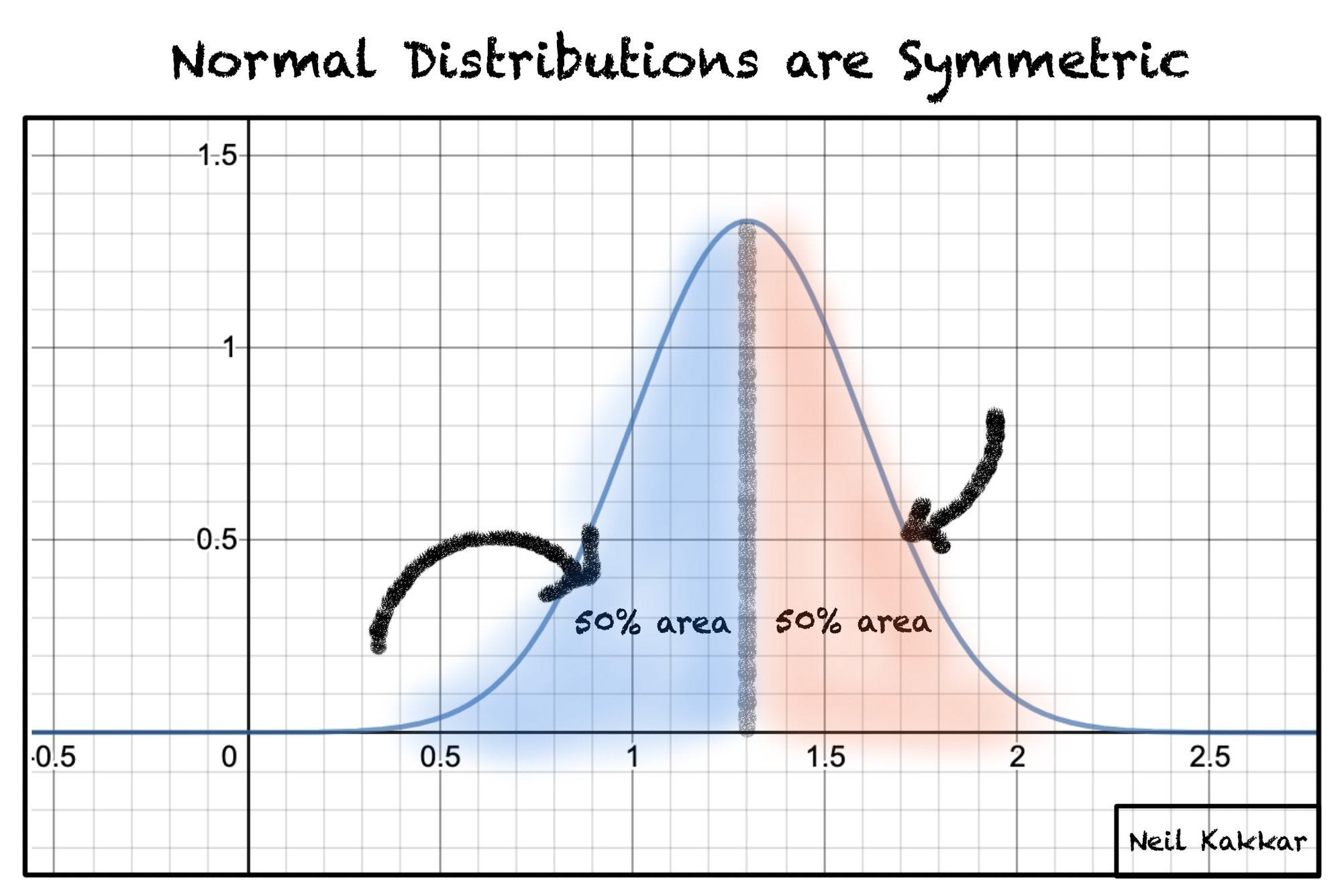Another important property is that we don’t need a lot of information to describe a normal distribution.

Indeed, we only need two things:

1. The mean. Most people just call this “the average.” It’s what you get if you add up the value of all your observations, then divide that number by the number of observations. For example, the average of these three numbers: `1, 2, 3 = (1 + 2 + 3) / 3 = 2`
2. The standard deviation. This tells you how rare an observation would be. Most observations fall within one standard deviation of the mean. Fewer observations are two standard deviations from the mean. And even fewer are three standard deviations away (or further).

Together, the mean and the standard deviation make up everything you need to know about a distribution.

#critical-thinking #science #statistics #math #data-science

## Buddha Community1603963200

## 68–95–99 Rule — Normal Distribution Explained in Plain English

Meet Mason. He’s an average American 40-year-old: 5 foot 10 inches tall and earning \$47,000 per year before tax.

How often would you expect to meet someone who earns 10x as much as Mason?

And now, how often would you expect to meet someone who is 10x as tall as Mason?

Your answers to the two questions above are different, because the distribution of data is different. In some cases, 10x above average is common. While in others, it’s not common at all.

## So what are normal distributions?

Today, we’re interested in normal distributions. They are represented by a bell curve shape, with a peak in the middle that tapers towards each edge. A lot of things follow this distribution, like your height, weight, and even IQ.

This distribution is exciting because it’s symmetric — which makes it easy to work with. You can reduce lots of complicated mathematics down to a few rules of thumb, because you don’t need to worry about weird edge cases.

For example, the peak always divides the distribution in half. There’s equal mass before and after the peak.Another important property is that we don’t need a lot of information to describe a normal distribution.

Indeed, we only need two things:

1. The mean. Most people just call this “the average.” It’s what you get if you add up the value of all your observations, then divide that number by the number of observations. For example, the average of these three numbers: `1, 2, 3 = (1 + 2 + 3) / 3 = 2`
2. The standard deviation. This tells you how rare an observation would be. Most observations fall within one standard deviation of the mean. Fewer observations are two standard deviations from the mean. And even fewer are three standard deviations away (or further).

Together, the mean and the standard deviation make up everything you need to know about a distribution.

#critical-thinking #science #statistics #math #data-science1624298520

## Introduction

In a series of weekly articles, I will be covering some important topics of statistics with a twist.

The goal is to use Python to help us get intuition on complex concepts, empirically test theoretical proofs, or build algorithms from scratch. In this series, you will find articles covering topics such as random variables, sampling distributions, confidence intervals, significance tests, and more.

At the end of each article, you can find exercises to test your knowledge. The solutions will be shared in the article of the following week.

Articles published so far:

As usual, the code is available on my GitHub.

#statistics #distribution #python #machine-learning #sampling distributions with python #sampling distributions1621248900

## What is Database Normalization in SQL Server – MS SQL Server – Zero to Hero Query Master

What is Database Normalization

Database normalization is the step by step process of organizing data to minimize data redundancy i.e. Data duplication which in turn ensures data consistency

• Normalization is a database design technique that reduces data redundancy and eliminates undesirable characteristics like Insertion, Update and Deletion Anomalies.
• Normalization rules divide larger tables into smaller tables and link them using relationships.
• The purpose of Normalization in SQL is to eliminate redundant (repetitive) data and ensure data is stored logically.
• The inventor of the relational model Edgar Codd proposed the theory of normalization of data with the introduction of the First Normal Form, and he continued to extend theory with Second and Third Normal Form. Later he joined Raymond F. Boyce to develop the theory of Boyce-Codd Normal Form.

#sql server #1nf #2nf #3nf #4nf #5nf #6nf #data #database in sql server #normalization #normalization forms #normalization in database #what is data1596896940

## Stop testing for normality

I see a lot of data scientists using tests such as the Shapiro-Wilk test and the Kolmogorov–Smirnov to test for normality. Stop doing this. Just stop. If you’re not yet convinced (and I don’t blame you!), let me show you why these are a waste of your time.

# Why do we care about normality?

We should care about normality. It’s an important assumption that underpins a wide variety of statistical procedures. We should always be sure of our assumptions and make efforts to check that they are correct. However, normality tests are not the way for us to do this.

However, in large samples (n > 30) which most of our work as data scientists is based upon the Central Limit Theorem usually applies and we need not worry about the normality of our data. But in cases where it does not apply let’s consider how we can check for normality in a range of different samples.

# Normality testing in small samples

First let us consider a small sample. Say n=10. Let’s look at the histogram for this data.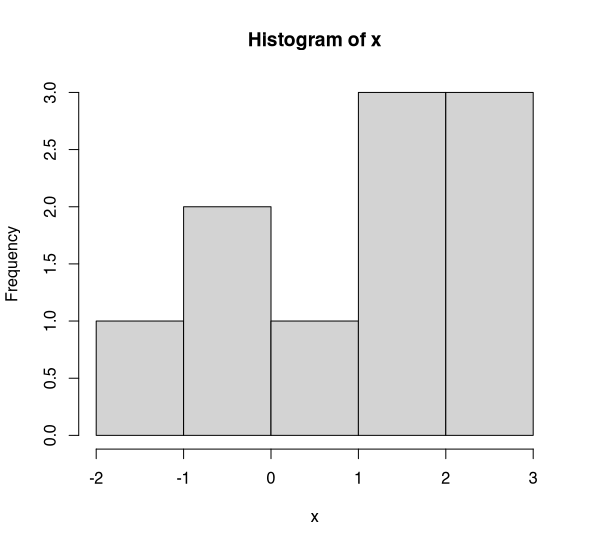Histogram of x (n=10). (Image by author)

Is this normally distributed? Doesn’t really look like it — does it? Hopefully you’re with me and accept that this isn’t normally distributed. Now let’s perform the Shapiro-Wilk test on this data.

Oh. p=0.53. No evidence to suggest that x is not normally distributed. Hmm. What do you conclude then. Well, of course, not being evidence that x is not normally distributed does not mean that x is normally distributed. What’s actually happening is that in small samples the tests are _underpowered _to detect deviations from normality.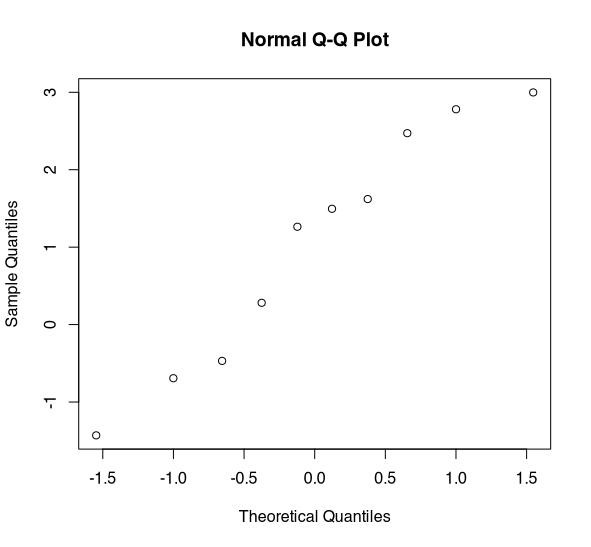Normal Q-Q Plot of x (n=10). (Image by author)

The best way to assess normality is through the use of a quantile-quantile plot — Q-Q plot for short. If the data is normally distributed we would expect to see a straight line. This data shows some deviation from normality, the line is not very straight. There appears to be some issues in the tail. Admittedly, without more data it is hard to say.

With this data, I would have concerns about assuming normality as there appears to be some deviation in the Q-Q plot and in the histogram. But, if we had just relied on our normality test, we wouldn’t have picked this up. This is because the test is underpowered in small samples.

# Normality testing in large samples

Now let’s take a look at normality testing in a large sample (n=5000). Let’s take a look at a histogram.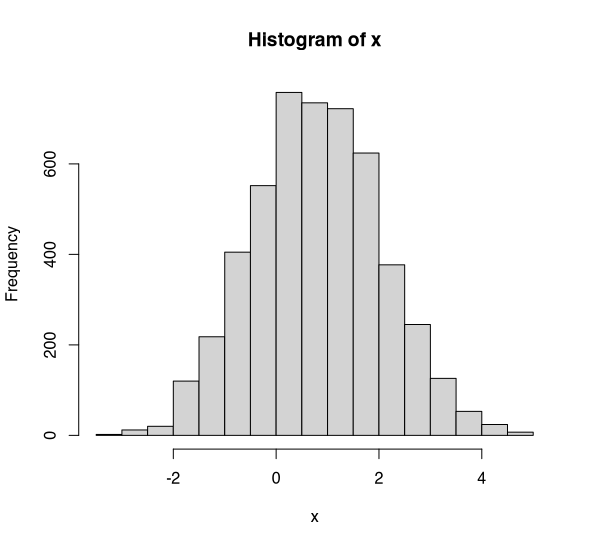Histogram of x (n=5000). (Image by author)

I hope you’d all agree that this looks to be normally distributed. Okay, so what does the Shapiro-Wilk test say. Bazinga! p=0.001. There’s very strong evidence that x is not normally distributed. Oh dear. Well, let’s take a quick look at our Q-Q plot. Just to double check.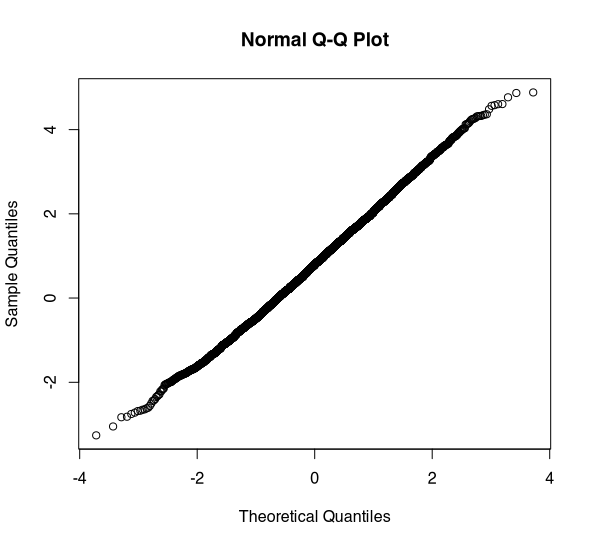Normal Q-Q plot for x (n=5000). (Image by author)

Wow. This looks to be normally distributed. In fact, there shouldn’t be any doubt that this is normally distributed. But, the Shapiro-Wilk test says it isn’t.

What’s going on here? Well the Shapiro-Wilk test (and other normality tests) are designed to test for theoretical normality (i.e. the perfect bell curve). In small samples these tests are underpowered to detect quite major deviations from normality which can be easily detected through graphical methods. In larger samples these tests will detect even extremely minor deviations from theoretical normality that are not of practical concern.

# Conclusion

Hopefully, I have shown you that normality tests are not of practical utility for data scientists. Don’t use them. Forget about them. At best, they are useless; at worst, they are misleading. If you want to assess the normality of some data, use Q-Q plots and histograms. They’ll give you a much clearer picture about the normality of your data.

#normal-distribution #statistics #tests-of-normality #mathematics #data-science1623263280

## Data Distribution in Apache Ignite

This blog is an abridged version of the talk that I gave at the Apache Ignite community meetup. You can download the slides that I presented at the meetup here. In the talk, I explain how data in Apache Ignite is distributed.

### Why Do You Need to Distribute Anything at all?

Inevitably, the evolution of a system that requires data storage and processing reaches a threshold. Either too much data is accumulated, so the data simply does not fit into the storage device, or the load increases so rapidly that a single server cannot manage the number of queries. Both scenarios happen frequently.

Usually, in such situations, two solutions come in handy—sharding the data storage or migrating to a distributed database. The solutions have features in common. The most frequently used feature uses a set of nodes to manage data. Throughout this post, I will refer to the set of nodes as “topology.”

The problem of data distribution among the nodes of the topology can be described in regard to the set of requirements that the distribution must comply with:

1. Algorithm. The algorithm allows the topology nodes and front-end applications to discover unambiguously on which node or nodes an object (or key) is located.
2. Distribution uniformity. The more uniform the data distribution is among the nodes, the more uniform the workloads on the nodes is. Here, I assume that the nodes have approximately equal resources.
3. Minimal disruption. If the topology is changed because of a node failure, the changes in distribution should affect only the data that is on the failed node. It should also be noted that, if a node is added to the topology, no data swap should occur among the nodes that are already present in the topology.

#tutorial #big data #distributed systems #apache ignite #distributed storage #data distribution #consistent hashing